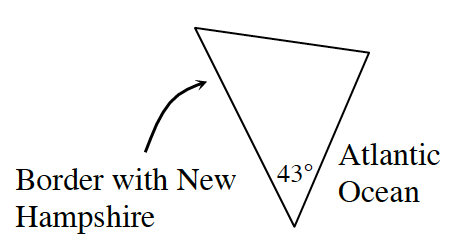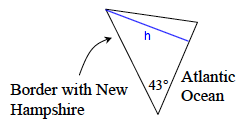### Home > GC > Chapter 9 > Lesson 9.2.4 > Problem9-92

9-92.

York County, Maine, is roughly triangular in shape. To help calculate its area, Sergio has decided to use a triangle to model the region, as shown at right. According to his map, the border with New Hampshire is $165$ miles long, while the coastline along the Atlantic Ocean is approximately $100$ miles long. If the angle at the tip of Maine is $43°$, as shown in the diagram, what is the approximate area of York County?Find the height of the triangle.

$\text{sin}(43°)=\frac{\text{opp}}{\text{hyp}}=\frac{h}{165}$

$h = 165 · \text{sin} (43°)$

Then use this to find the area of the triangle:

$\frac{1}{2}(100)(165 \text{ sin}(43°))$$\text{Area}\approx5626.5$ square miles